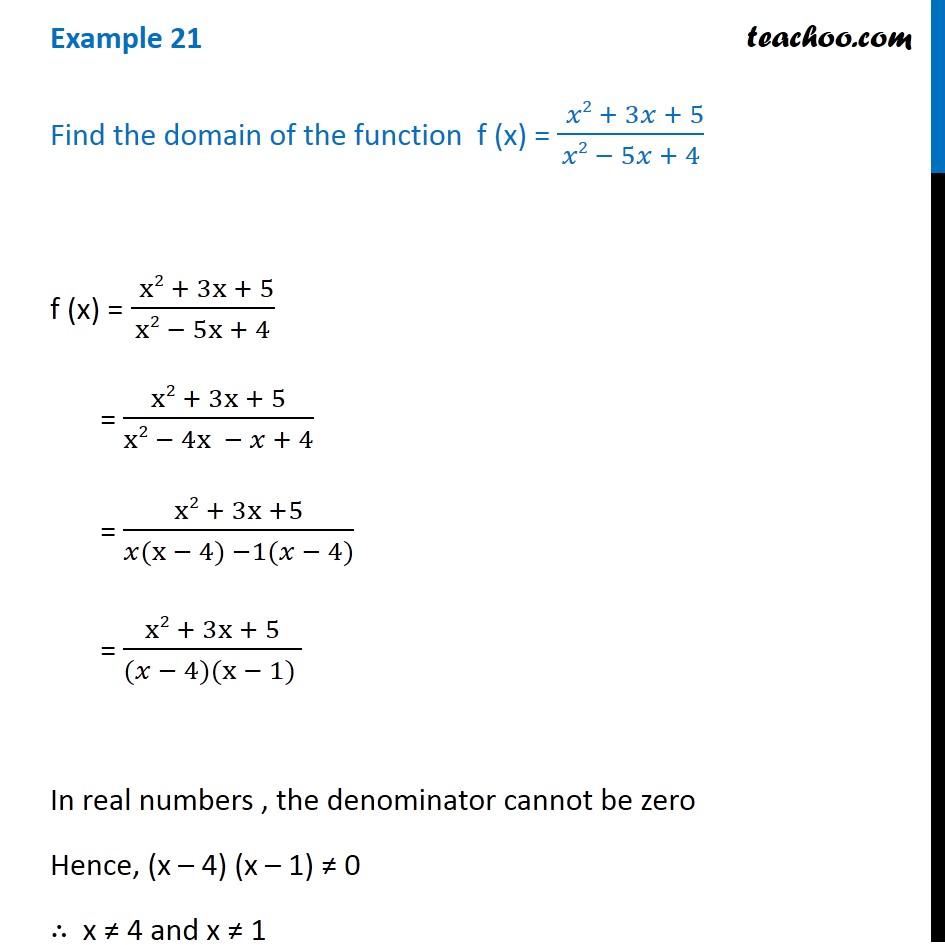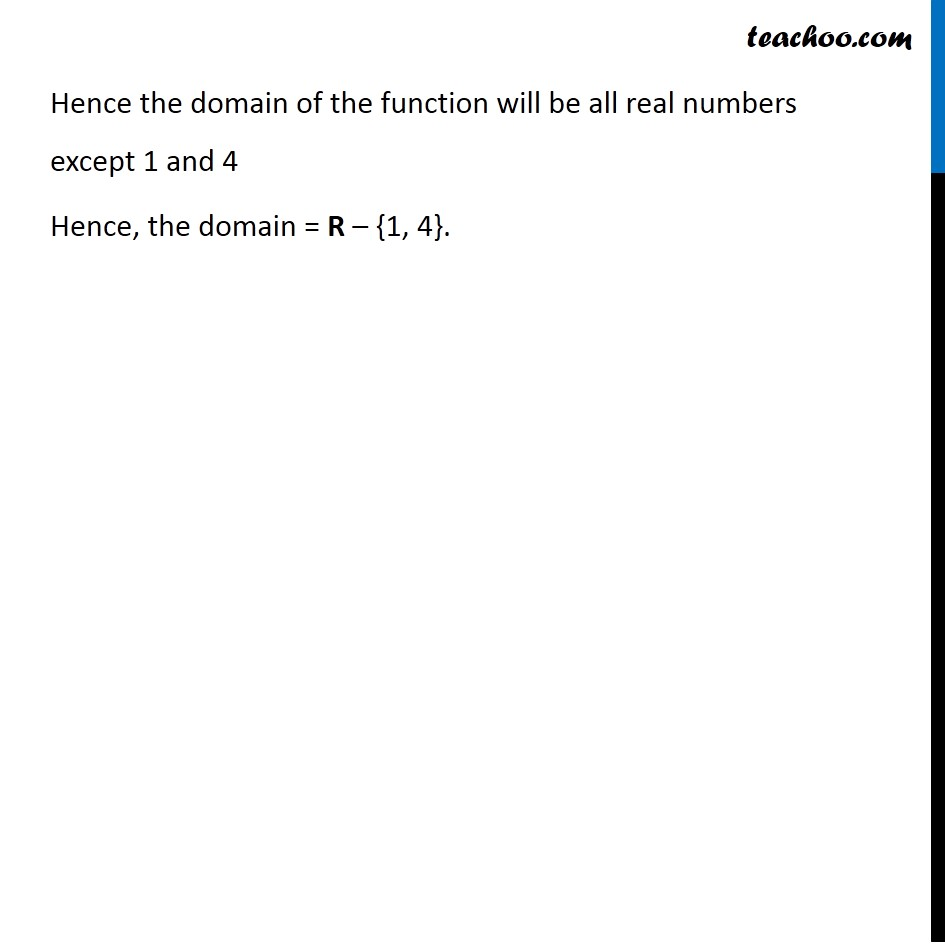1. Chapter 2 Class 11 Relations and Functions (Term 1)
2. Serial order wise
3. Examples

Transcript

Example 21 Find the domain of the function "f" (x) = (" " 2 + 3 + 5)/( 2 5 + 4) "f" (x) = (" " x2 + 3x + 5)/(x2 5x + 4) = (x2 + 3x + 5)/(x2 4x + 4) = (x2 + 3x +5)/( (x 4) 1( 4)) = (x2 + 3x + 5)/(( 4)(x 1) ) In real numbers , the denominator cannot be zero Hence (x 4) (x 1) 0 x 4 and x 1 Hence the domain of the function will be all real numbers except 1 and 4 Hence, the domain = R {1, 4}.

Examples

Chapter 2 Class 11 Relations and Functions (Term 1)
Serial order wise